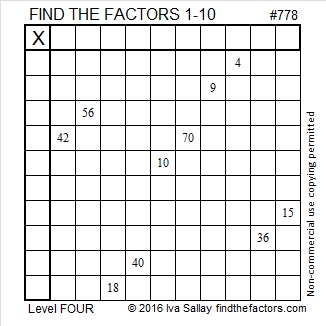# 778 and Level 4

• 778 is a composite number.
• Prime factorization: 778 = 2 x 389
• The exponents in the prime factorization are 1 and 1. Adding one to each and multiplying we get (1 + 1)(1 + 1) = 2 x 2 = 4. Therefore 778 has exactly 4 factors.
• Factors of 778: 1, 2, 389, 778
• Factor pairs: 778 = 1 x 778 or 2 x 389
• 778 has no square factors that allow its square root to be simplified. √778 ≈ 27.892651.Here’s today’s puzzle. Hints for solving it are in a table at the end of the post.Print the puzzles or type the solution on this excel file: 10-factors-2016

—————————————

OEIS.org informs us that 778 is the number of ways  a 1 x 5 rectangle can be surrounded by other 1 x 5 rectangles. I’m not going to publish or even find all 778 possible ways, but here is one of them:27² + 7² = 778.

We can use those numbers to compute a Pythagorean triple: 2(27)(7), 27² – 7², 27² + 7², which turns out to be 378-680-778, and thus 378² + 680² = 778².

778 is also the sum of three squares three different ways:

• 25² + 12² + 3² = 778
• 24² + 11² + 9² = 778
• 21² + 16² + 9² = 778

778 is palindrome 1G1 BASE 21 (G is 16 base 10)

That palindrome means that 1(21²) + 16(21) + 1(1) = 778.

—————————————## One thought on “778 and Level 4”

1.Joseph Nebus

You know, that’s a weird and surprising thing for there to be 778 of.

This site uses Akismet to reduce spam. Learn how your comment data is processed.